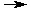Ionic Equations

## Ionic Equations

 1 Write the molecular equation and balance it. 2 AgNO3 + Na2SO4Ag2SO4 + 2 NaNO3 2 Determine the state of each substance (gas, liquid, solid, aqueous). Use the solubility rules to determine which of the ionic compounds are soluble in water. Soluble ionics are identified with an (aq), insoluble ones with an (s). Most elements and covalent compounds are insoluble in water and should be shown with an (s), (l) or (g). 2 AgNO3(aq) + Na2SO4(aq)Ag2SO4(s) + 2 NaNO3(aq) 3 Write the ionic equation by breaking all the soluble ionic compounds (those marked with an (aq)) into their respective ions. Each ion should be shown with its charge and an (aq) to show that it is present in solution. Use coefficients to show the number of each ion present. Rewrite the elements and covalent compounds as they appeared in the preceeding step. 2 Ag+(aq) + 2 NO3-(aq) + 2 Na+(aq) + SO4-2(aq)Ag2SO4(s) + 2 Na+(aq) + 2 NO3-(aq) 4 Write the net ionic equation by removing the spectator ions. Spectator ions are those ions that appear exactly the same on each side of the ionic equation. 2 Ag+(aq) + SO4-2(aq)Ag2SO4(s)